## Demo file

Below are some examples of different plotting styles to represent uncertainty in time series data. All plots are based on the th.mcmc data, the estimated posterior distributions of the volatility in daily returns from the Pound/Dollar exchange rate from 02/10/1981 to 28/6/1985. To run the demo file from your R console (ensure fanplot, zoo, tsbugs, RColorBrewer and colorspace packages are all installed beforehand). Note, tsbugs is no longer on CRAN - install with devtools::install_github("guyabel/tsbugs")

# if you want plots in separate graphic devices do not run this first line...
par(mfrow = c(10,2))
# run demo
demo(sv_fan, package = "fanplot", ask = FALSE)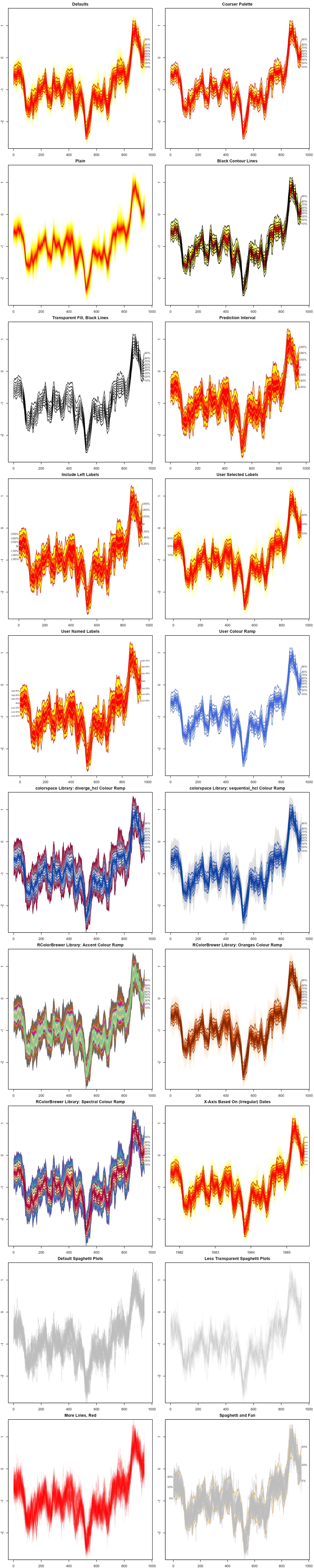Below is the code and the individual plots in the demo file

#### Main arguments

Default plot

library(fanplot)
# trim margins
par(mar=c(2,2,2,1.1))
# empty plot
plot(NULL, xlim = c(1, 965), ylim = range(th.mcmc)*0.85, main="Defaults")
fan(th.mcmc)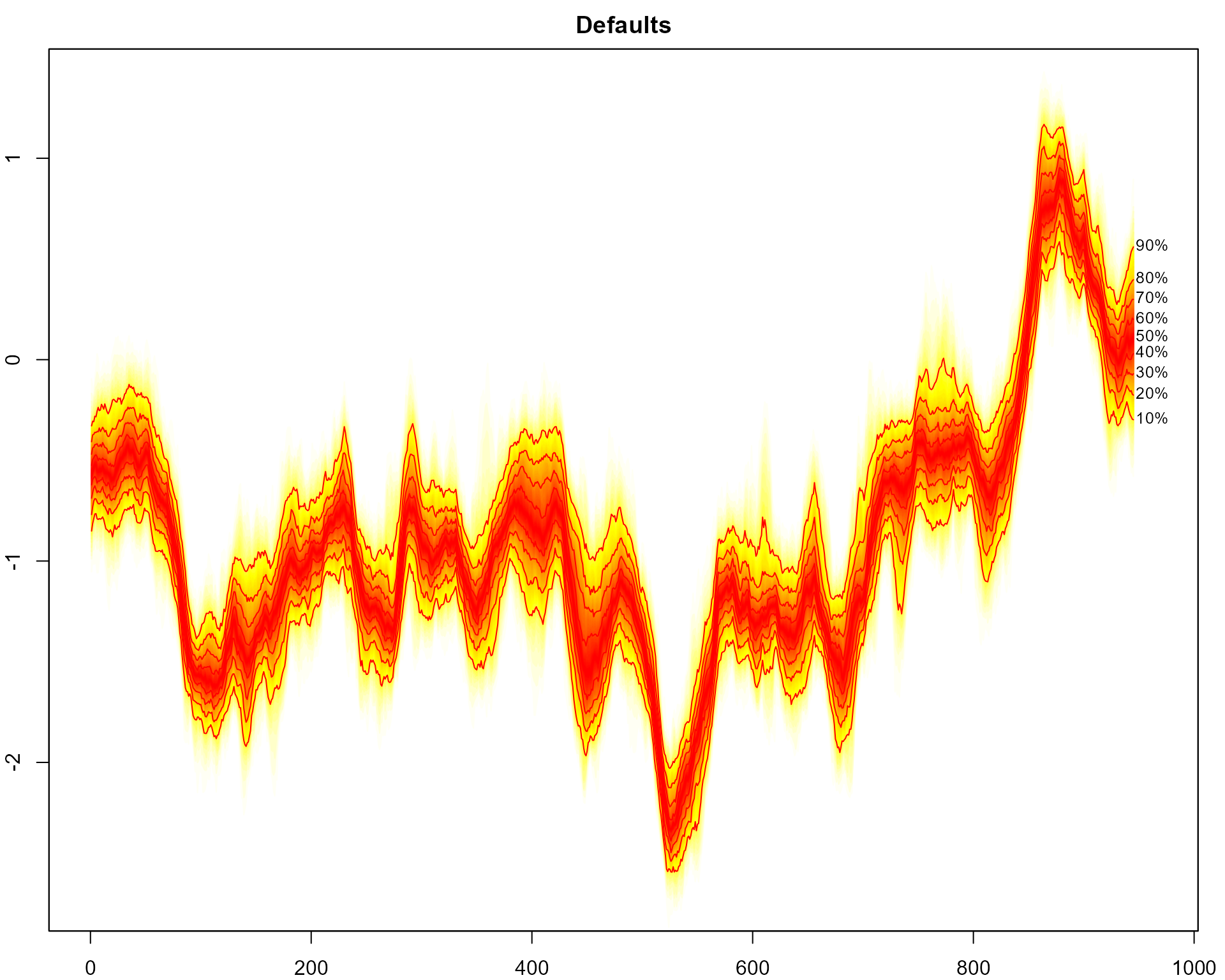Create a coarser fan

plot(NULL, xlim = c(1, 965), ylim = range(th.mcmc)*0.85, main="Coarser Palette")
fan(th.mcmc, probs = seq(10, 90, 10))Fan with no lines and text

plot(NULL, xlim = c(1, 965), ylim = range(th.mcmc)*0.85, main="Plain")
fan(th.mcmc, ln = NULL, rlab = NULL)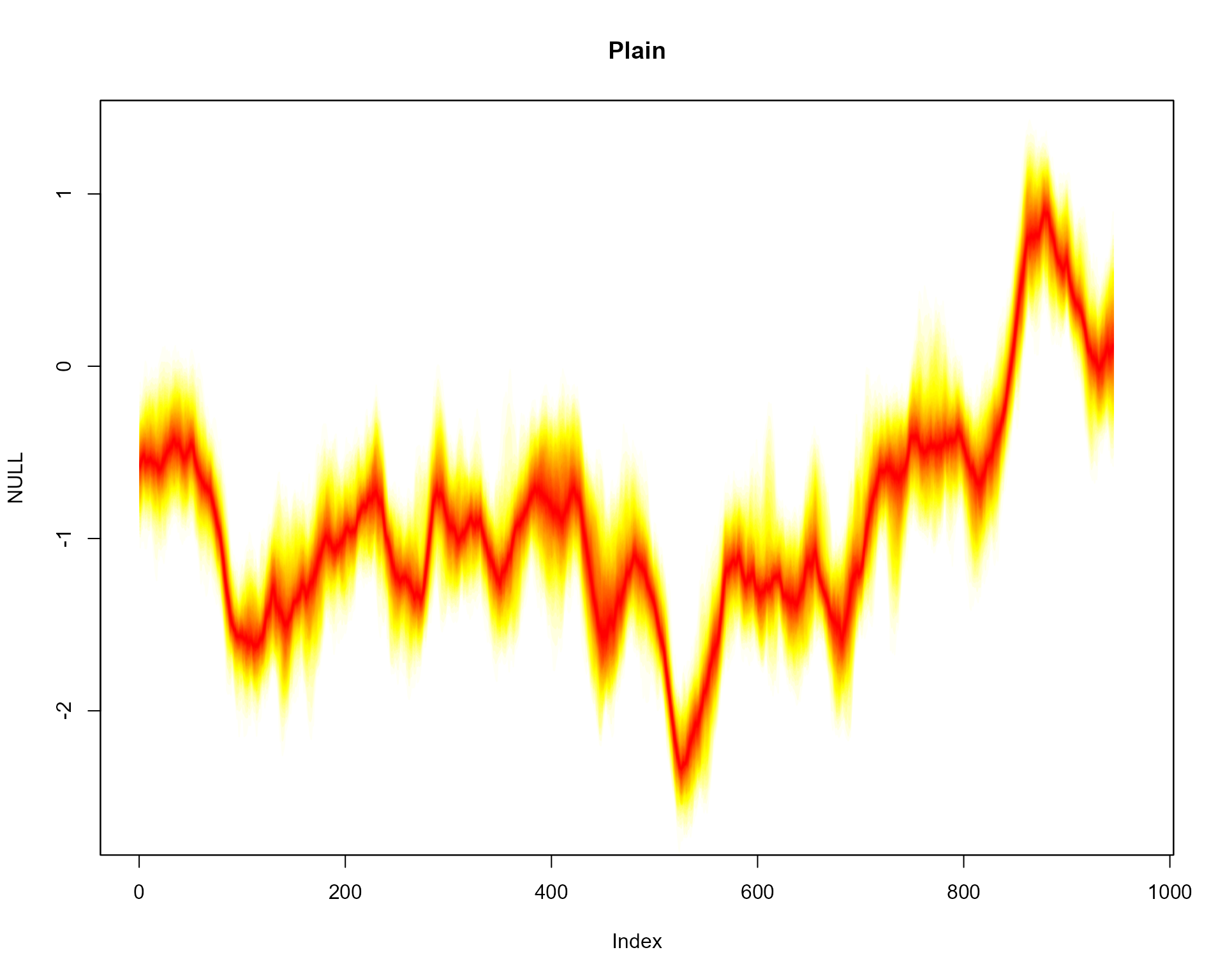Change contour line colours

plot(NULL, xlim = c(1, 965), ylim = range(th.mcmc)*0.85, main="Black Contour Lines")
fan(th.mcmc, probs = seq(10, 90, 10), ln.col = "black")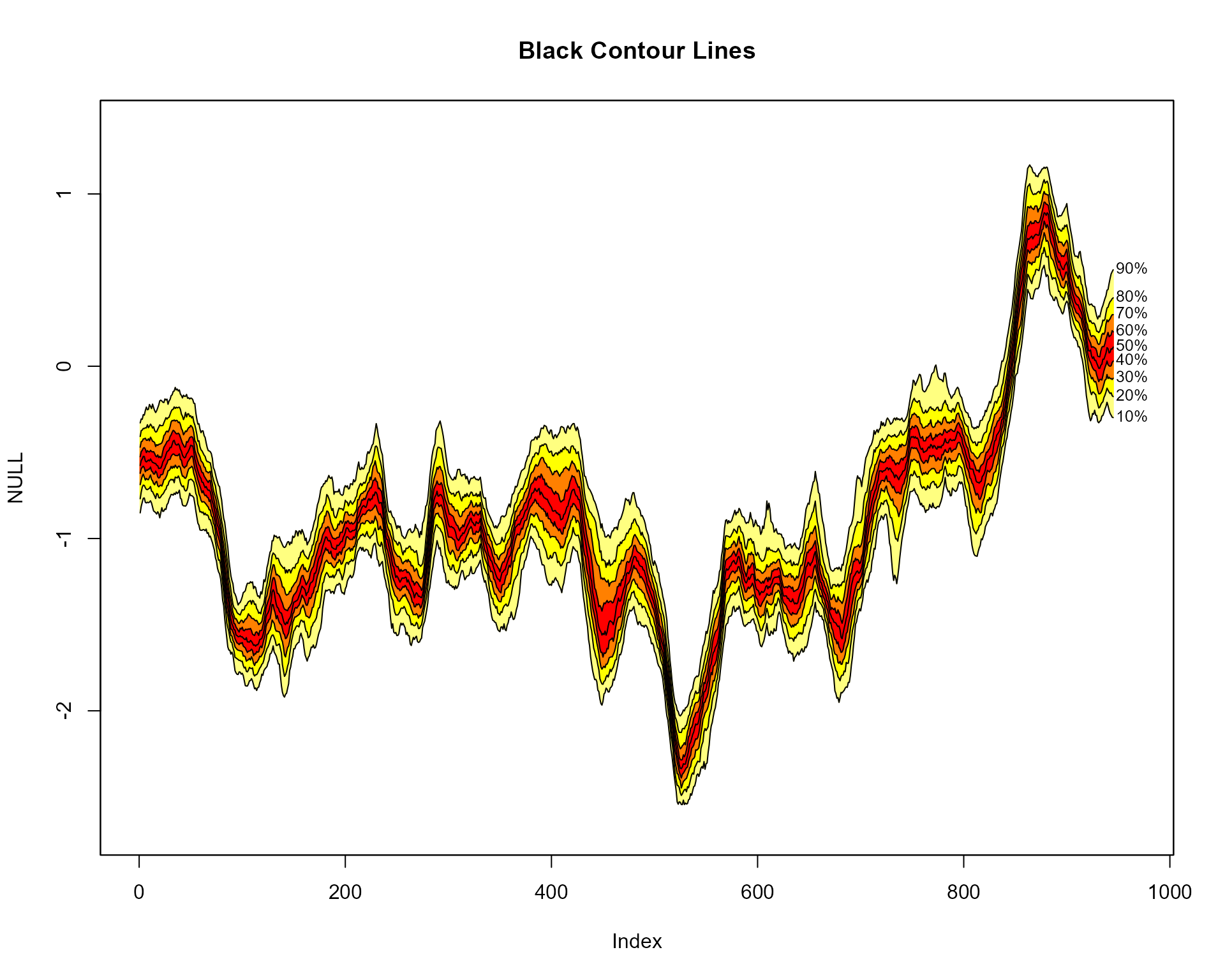Transparent fan fill to leave only percentile contour lines

plot(NULL, xlim = c(1, 965), ylim = range(th.mcmc)*0.85, main="Transparent Fill, Black Lines")
fan(th.mcmc, alpha=0, ln.col="black")Prediction intervals

plot(NULL, xlim = c(1, 985), ylim = range(th.mcmc)*0.85, main="Prediction Interval")
fan(th.mcmc, type = "interval")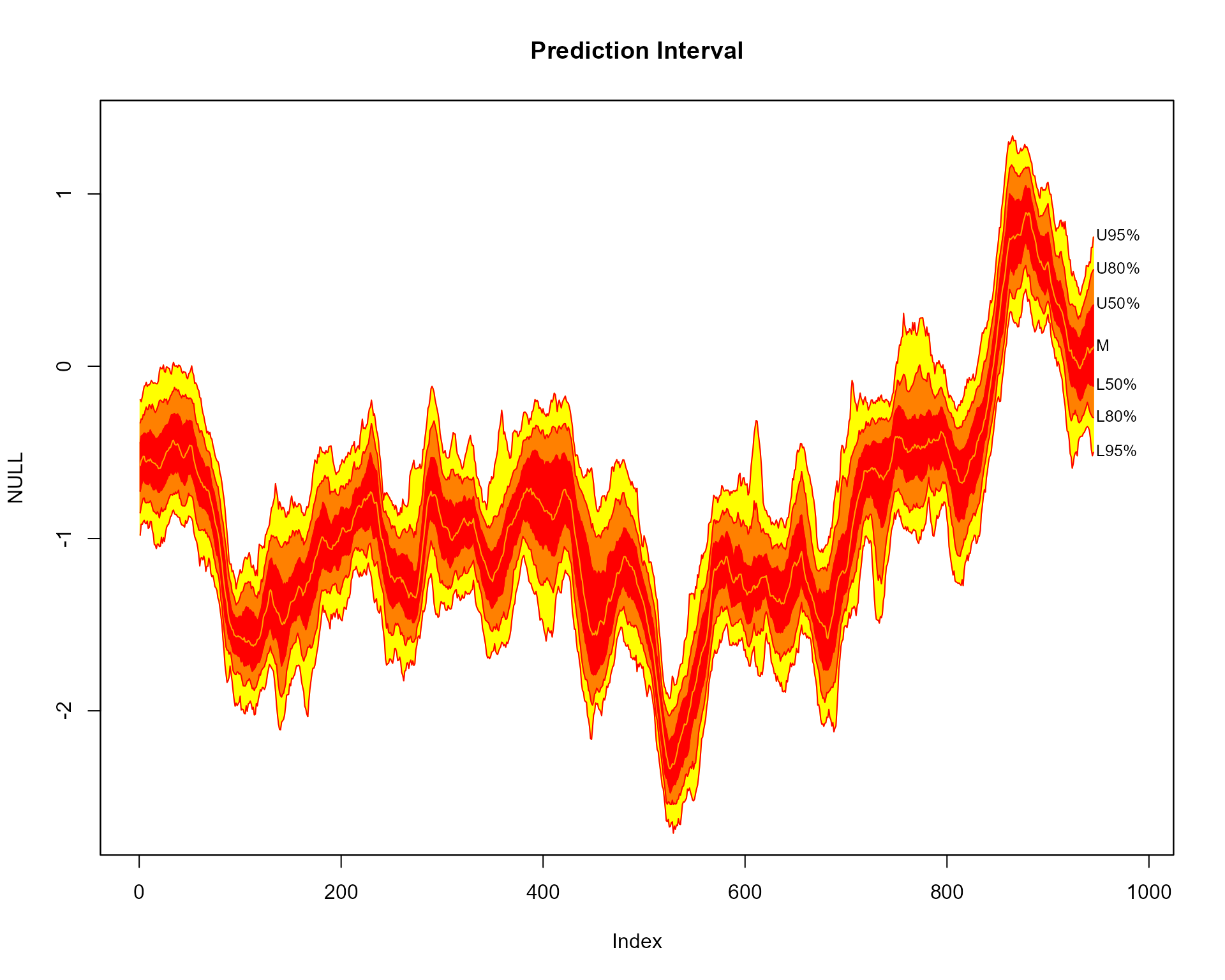#### Labels

Include left labels

plot(NULL, xlim = c(-40, 985), ylim = range(th.mcmc)*0.85, main="Include Left Labels")
fan(th.mcmc, type = "interval", llab = TRUE)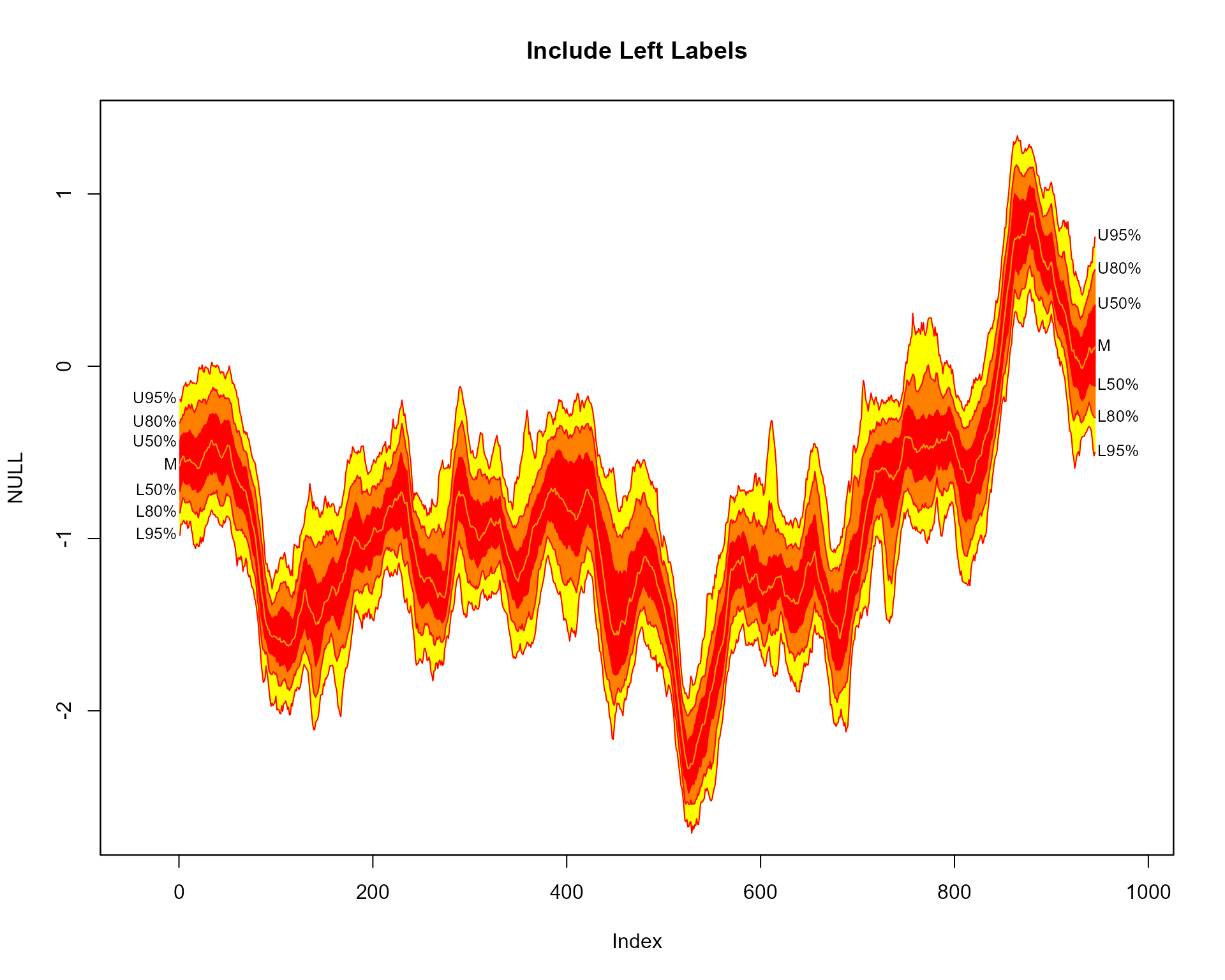Selected right labels

plot(NULL, xlim = c(-20, 965), ylim = range(th.mcmc)*0.85, main="User Selected Labels")
fan(th.mcmc, llab=c(0.1,0.5,0.9), rlab=c(0.2,0.5,0.8))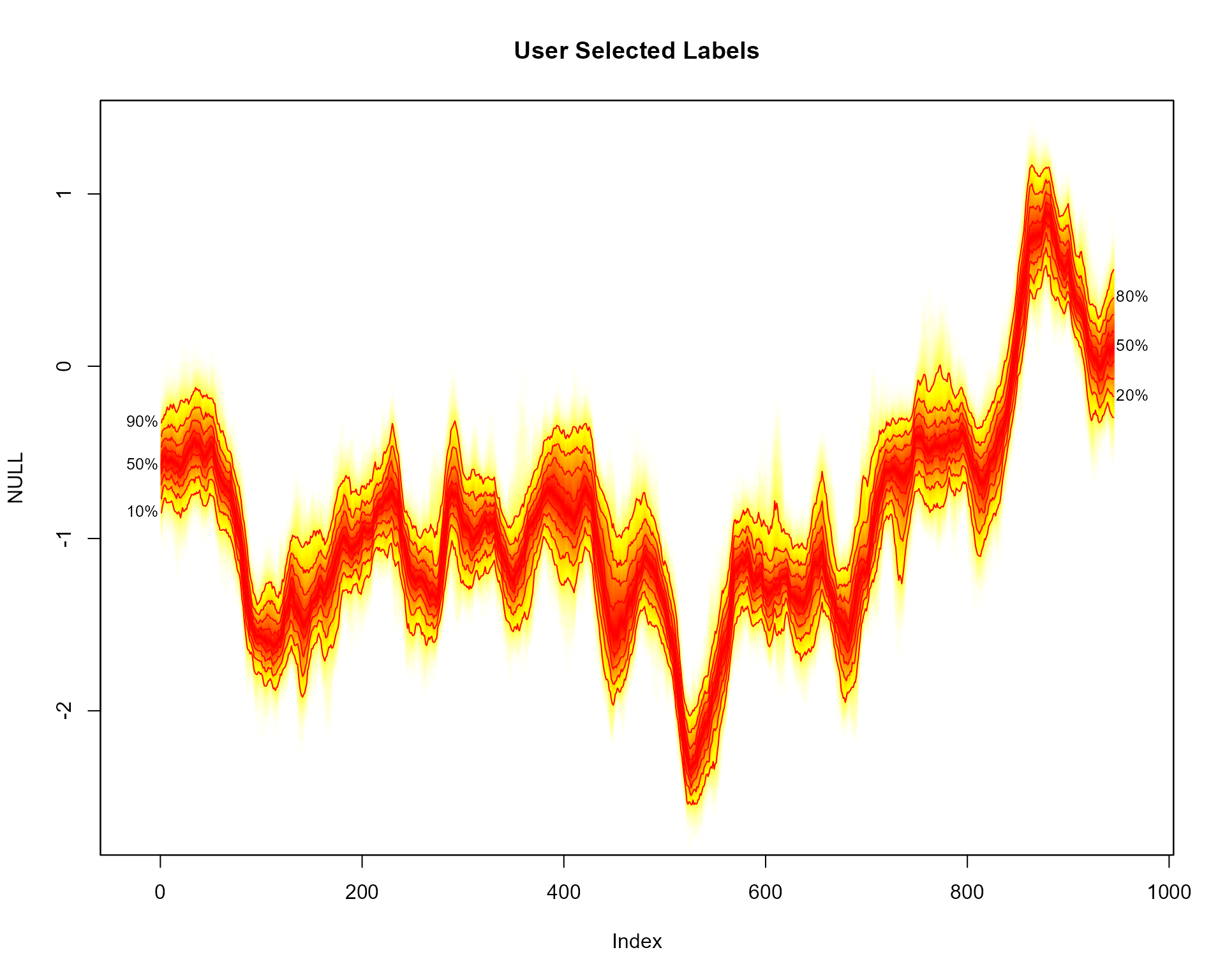Change prefixes of labels

plot(NULL, xlim = c(-50, 995), ylim = range(th.mcmc)*0.85, main="User Named Labels")
fan(th.mcmc, type = "interval", llab = TRUE, rcex = 0.6,
upplab = "Upp ", lowlab = "Low ", medlab="Med")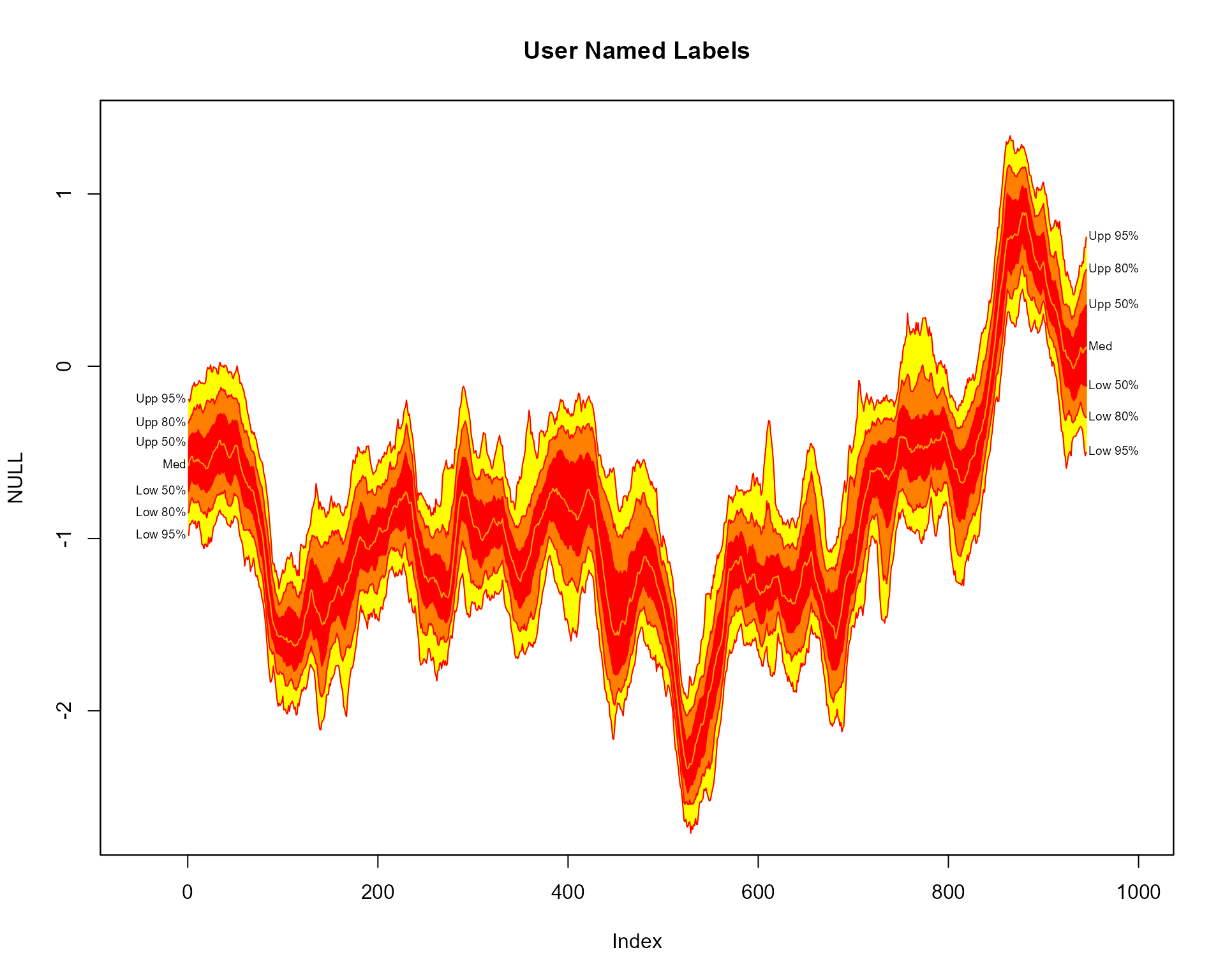#### Colour

Different colour scheme

plot(NULL, xlim = c(1, 965), ylim = range(th.mcmc)*0.85, main="User Colour Ramp")
fan(th.mcmc, probs = seq(10, 90, 10),
fan.col = colorRampPalette(c("royalblue", "grey", "white")))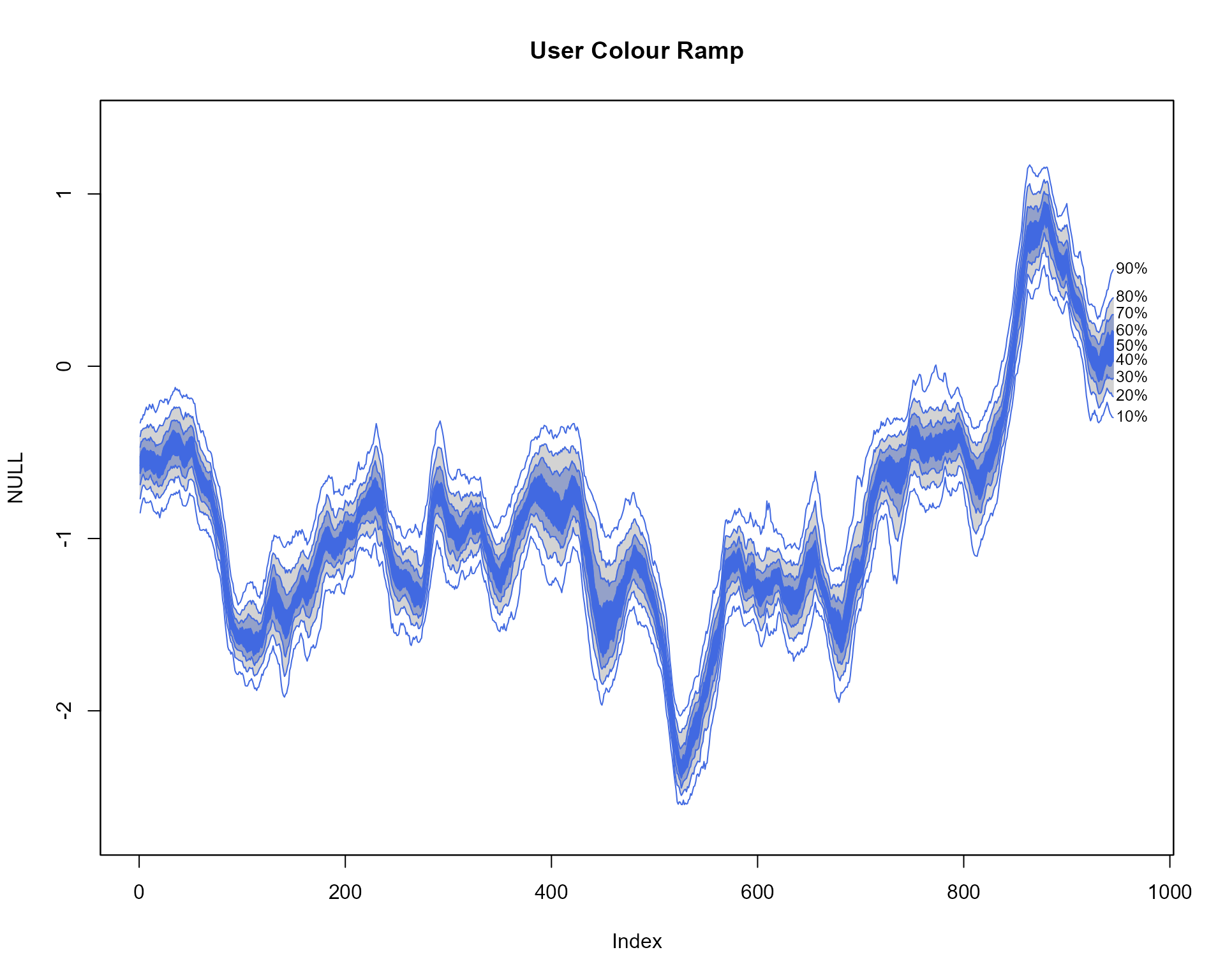Colours from the colorspace package

library(colorspace)
plot(NULL, xlim = c(0, 965), ylim = range(th.mcmc)*0.85, main="colorspace Library: diverge_hcl Colour Ramp")
fan(data = th.mcmc, fan.col = diverge_hcl)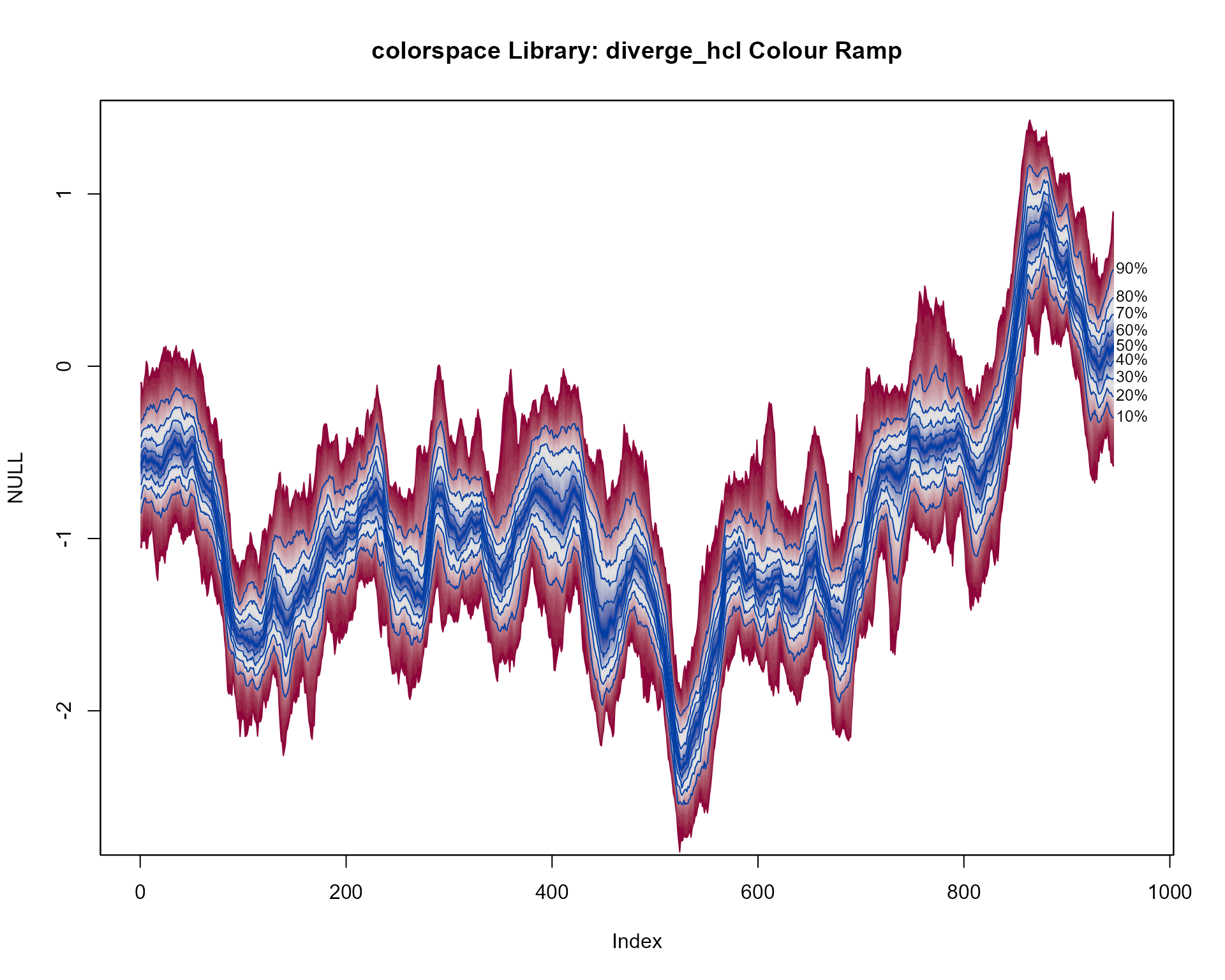Second colorspace colours example

plot(NULL, xlim = c(0, 965), ylim = range(th.mcmc)*0.85, main="colorspace Library: sequential_hcl Colour Ramp")
fan(data = th.mcmc, fan.col = sequential_hcl )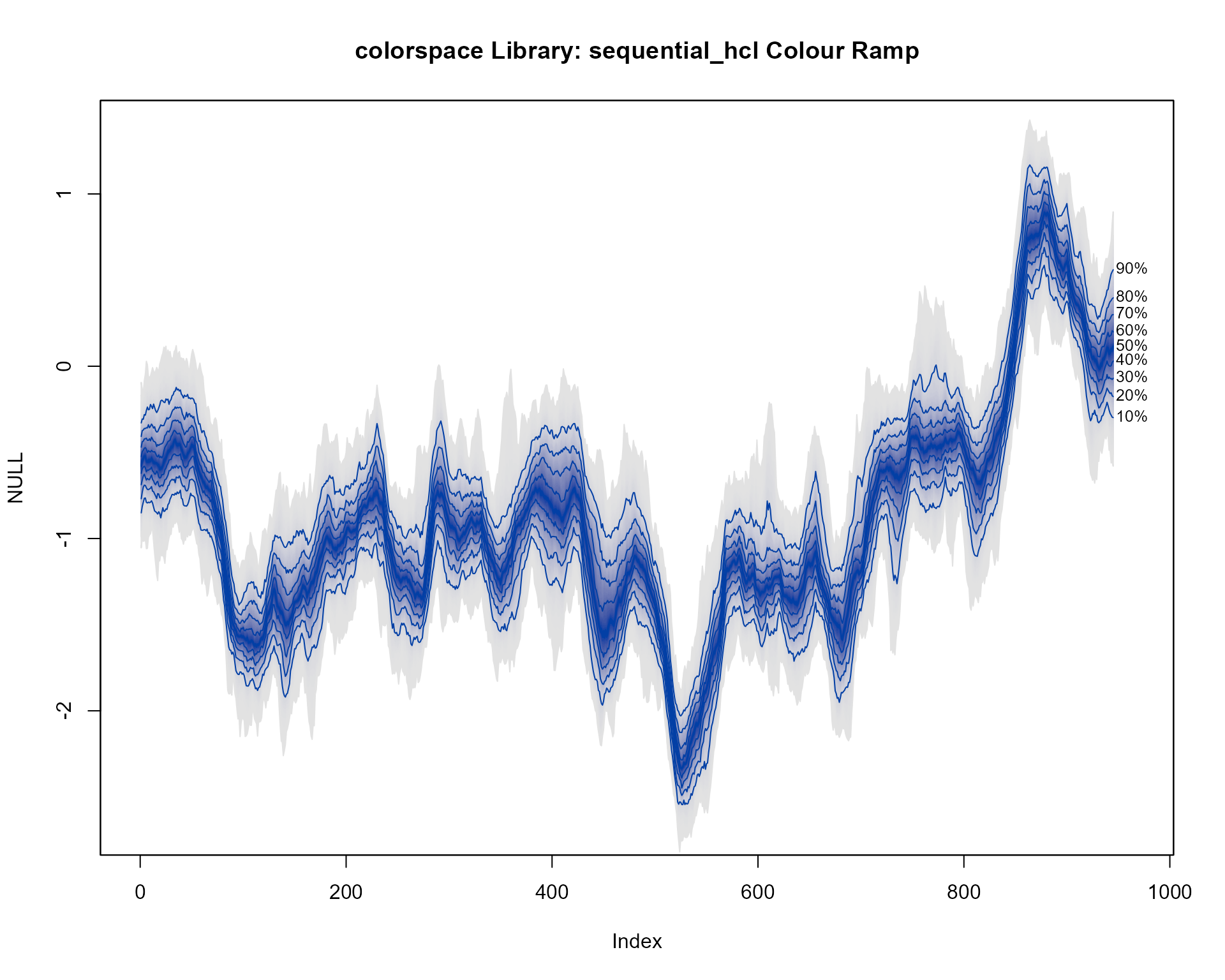Colours from the RColorBrewer package

library(RColorBrewer)
plot(NULL, xlim = c(0, 965), ylim = range(th.mcmc)*0.85, main="RColorBrewer Library: Accent Colour Ramp")
fan(data = th.mcmc,
fan.col = colorRampPalette(colors = brewer.pal(8,"Accent")) )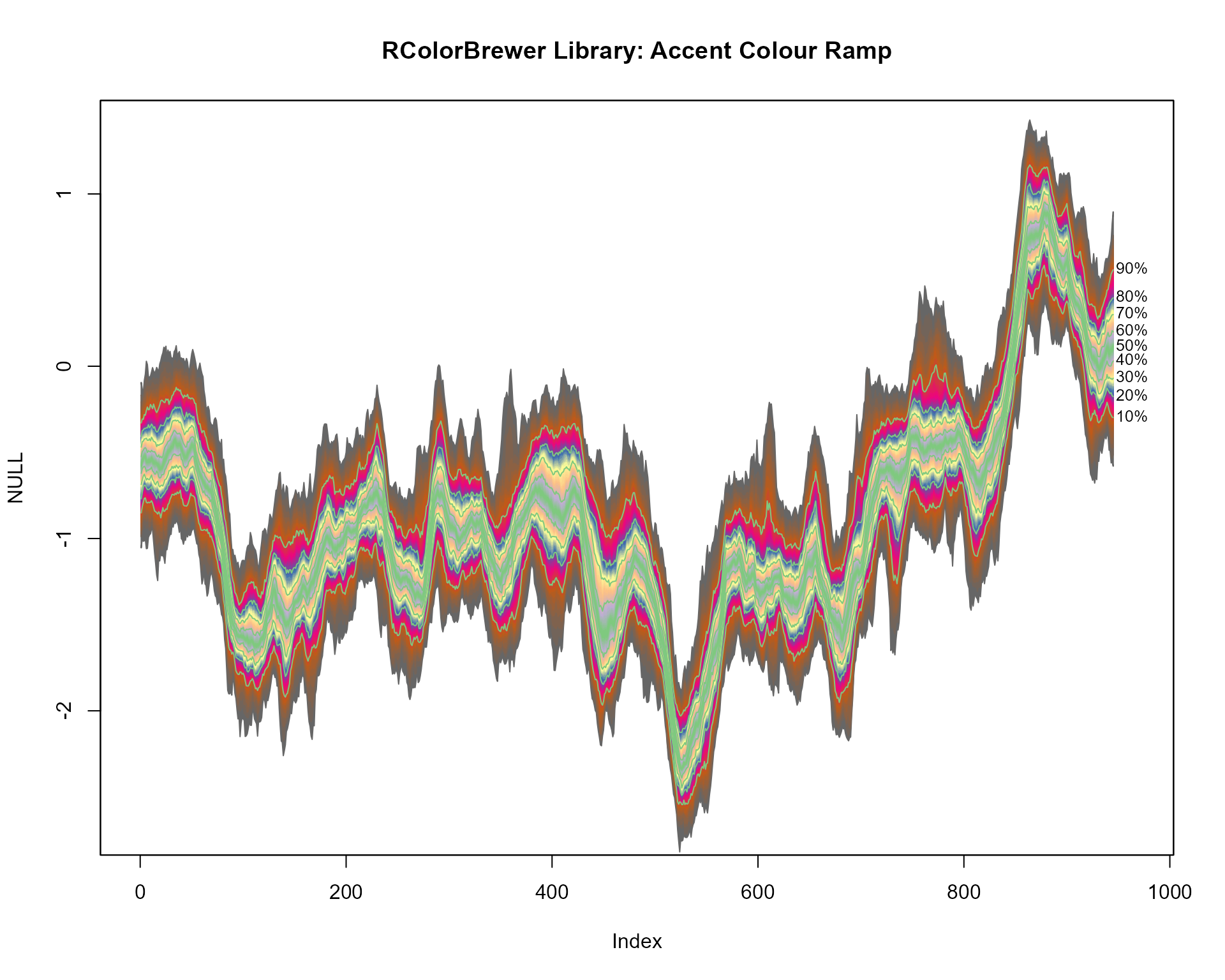Second RColorBrewer example

plot(NULL, xlim = c(0, 965), ylim = range(th.mcmc)*0.85, main="RColorBrewer Library: Oranges Colour Ramp")
fan(data = th.mcmc,
fan.col = colorRampPalette(colors = rev(brewer.pal(9,"Oranges"))) )Third RColorBrewer example

plot(NULL, xlim = c(0, 965), ylim = range(th.mcmc)*0.85, main="RColorBrewer Library: Spectral Colour Ramp")
fan(data = th.mcmc,
fan.col = colorRampPalette(colors = brewer.pal(11,"Spectral")) )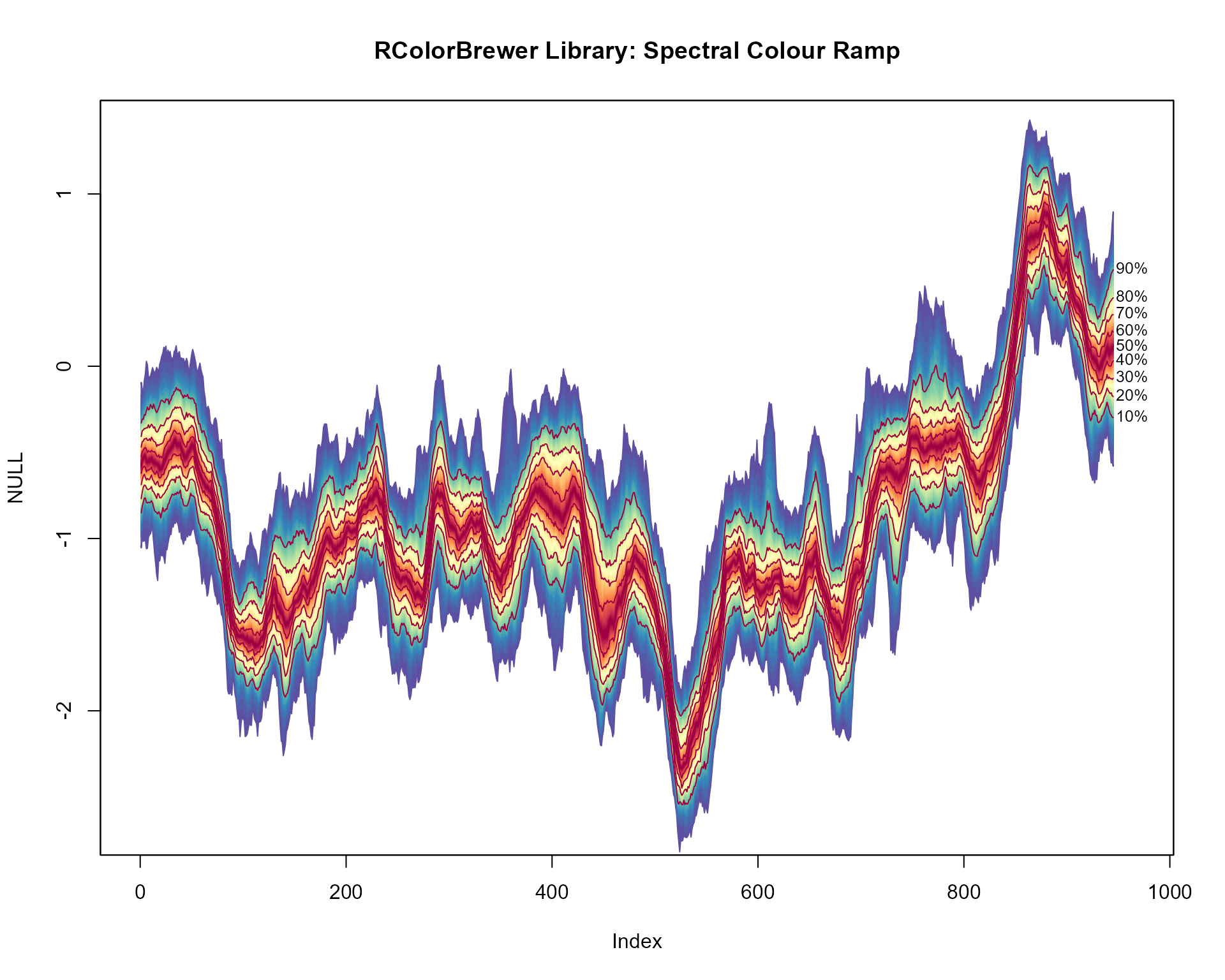#### Irregular time series

library(zoo)
th.mcmc2 <- zoo(th.mcmc, order.by=svpdx\$date)
plot(th.mcmc2[,1], type="n", ylim = range(th.mcmc)*0.85, main="X-Axis Based On (Irregular) Dates")
fan(data = th.mcmc2, rcex=0.5)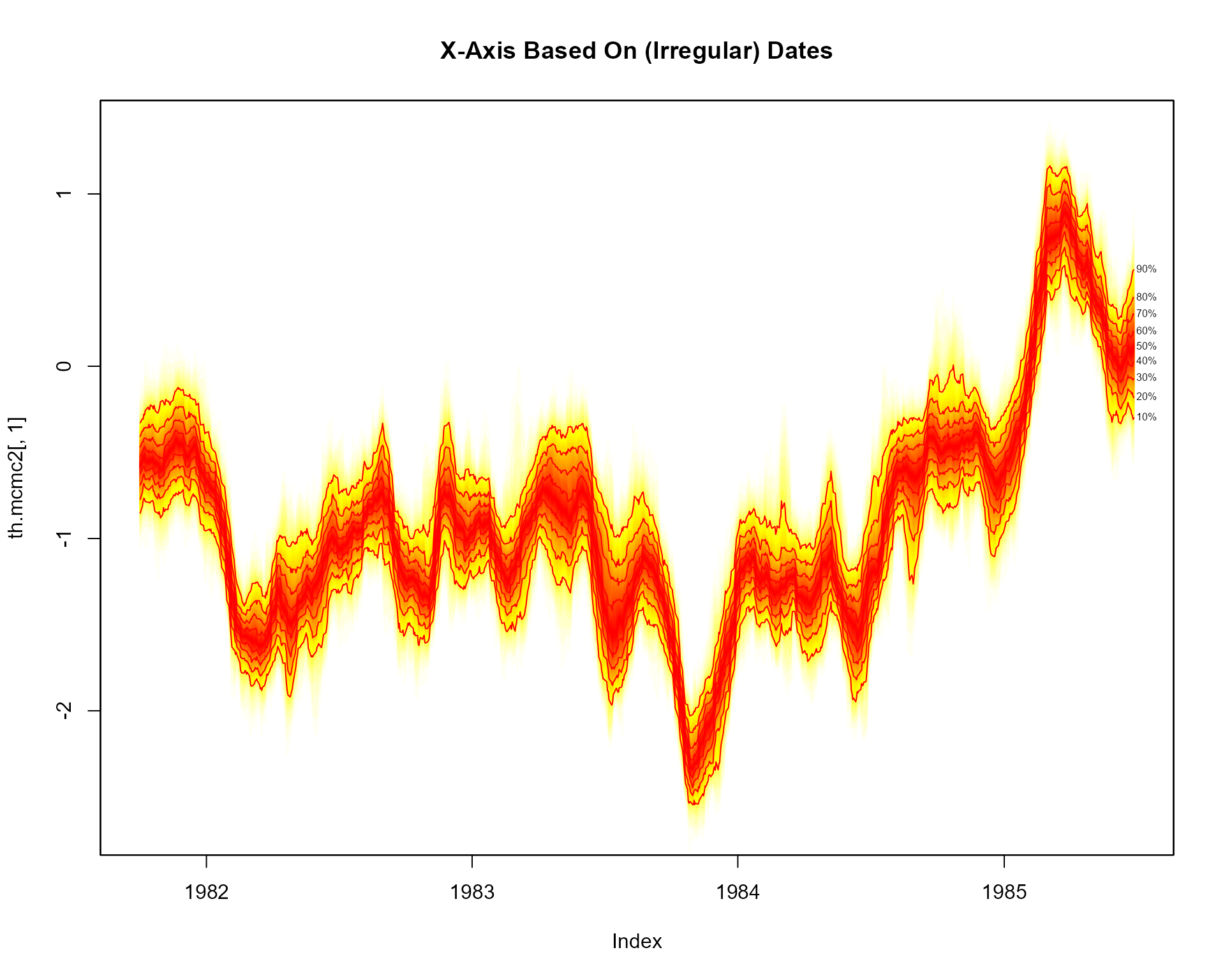#### Spaghetti plot

plot(NULL, xlim = c(1, 965), ylim = range(th.mcmc)*0.85, main="Default Spaghetti Plots")
fan(th.mcmc, style = "spaghetti")More transparency in lines

plot(NULL, xlim = c(1, 965), ylim = range(th.mcmc)*0.85, main="Less Transparent Spaghetti Plots")
fan(th.mcmc, style = "spaghetti", alpha = 0.1)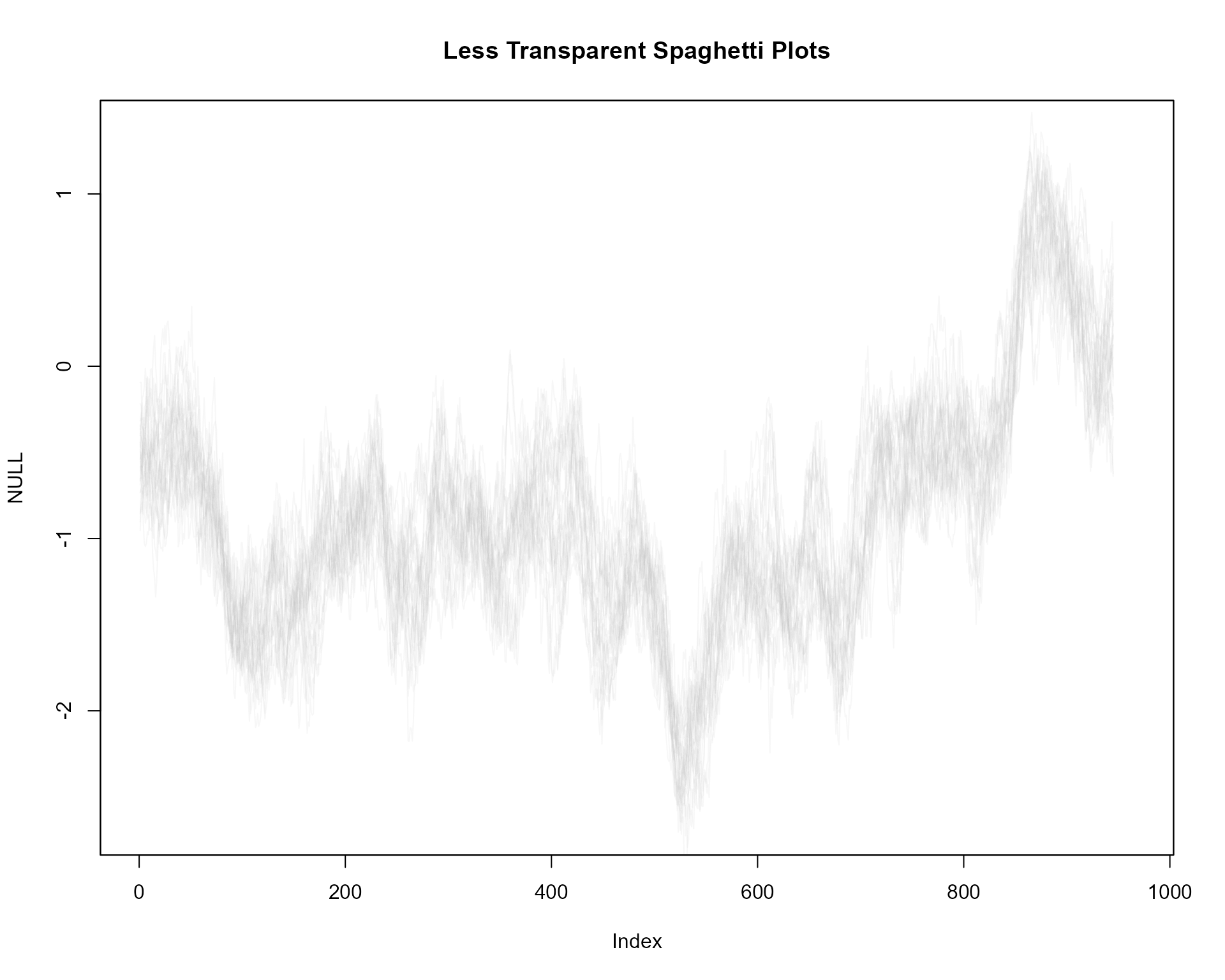More lines, red

plot(NULL, xlim = c(1, 965), ylim = range(th.mcmc)*0.85, main="More Lines, Red")
fan(th.mcmc, style = "spaghetti", ln.col = "red", n.spag = 100, alpha = 0.1)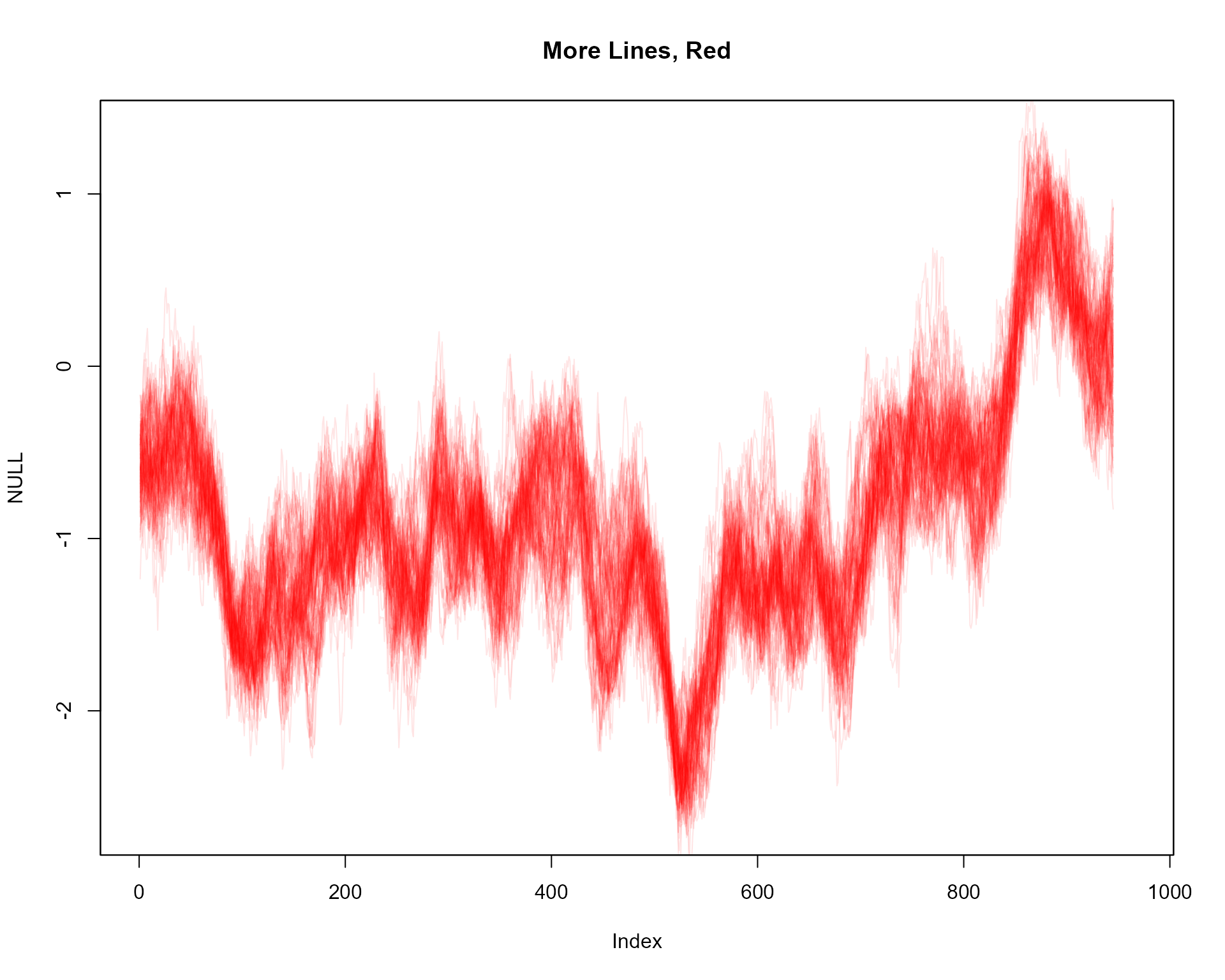#### Overlaying fans

Overlay spaghetti on transparent fan

plot(NULL, xlim = c(-20, 965), ylim = range(th.mcmc)*0.85, main="Spaghetti and Fan")
# transparent fan with visible lines
fan(th.mcmc, ln=c(5, 50, 95), llab=TRUE, alpha=0, ln.col="orange" )
# spaghetti lines
fan(th.mcmc, style="spaghetti")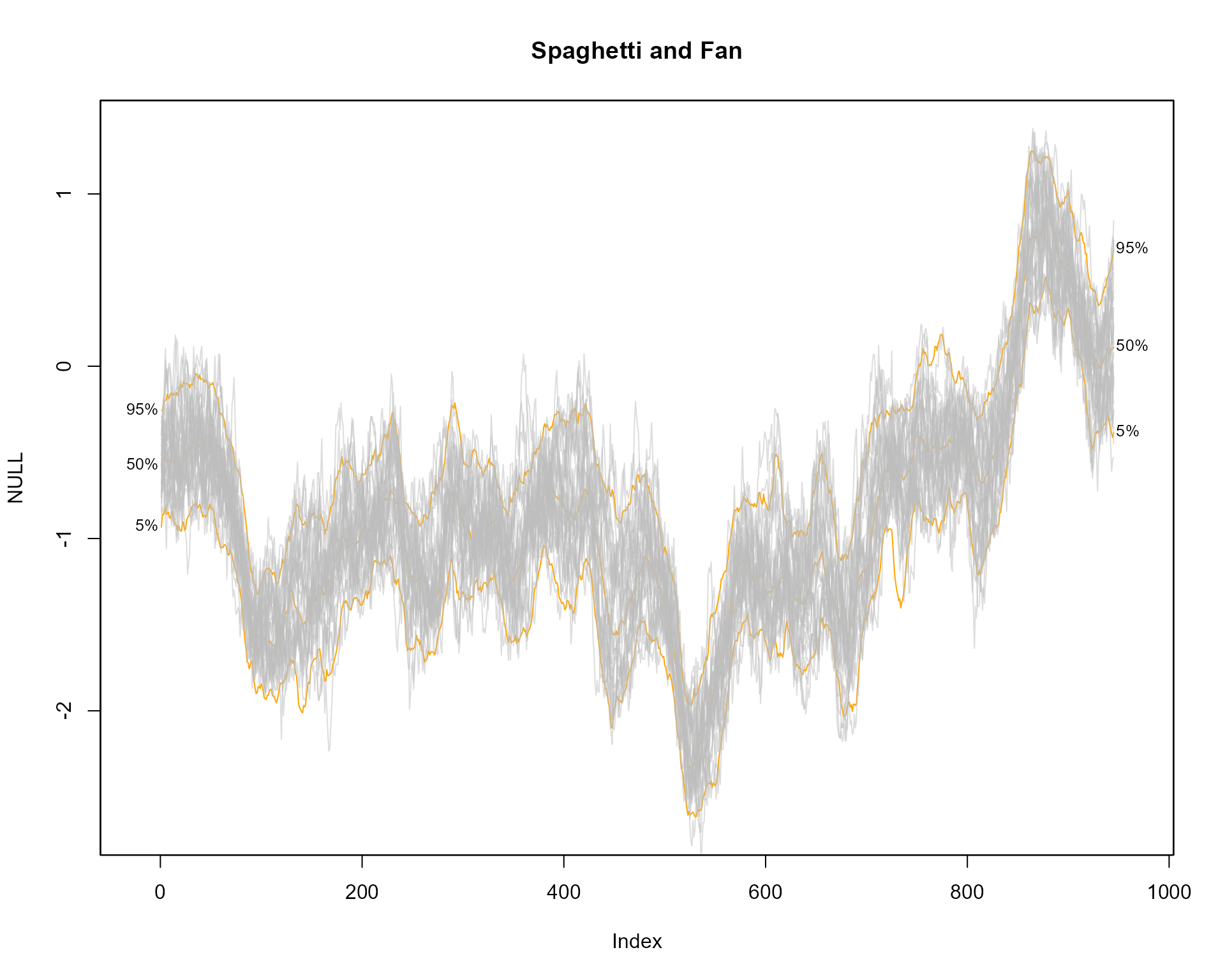#### Saving

Save as png

# dev.copy(png, file = "svplots.png", width=10, height=50, units="in", res=400)
# dev.off()